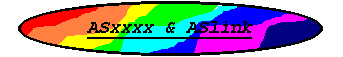## ASST8 Assembler#### ```ST8 REGISTER SET The following is a list of the ST8 registers used by ASST8: a - 8-bit accumulator xl,yl - LSB of index registers xh,yh - MSB of index registers x,y - 16-Bit index registers sp - 16-bit stack pointer cc - 8-bit condition code register ST8 INSTRUCTION SET The following list specifies the format for each addressing mode supported by ASST8: reg register addressing (a,x,xl,xh,y,yl,yh,sp,cc) #data immediate data *saddr 8-bit zero page addressing 0x00 <= saddr <= 0xFF laddr 16-bit addressing 0x0000 <= laddr <= 0xFFFF eaddr 24-bit addressing 0x000000 <= eaddr <= 0xFFFFFF (x) register indirect addressing (*sofst,r) short indexed (r = x,y,sp) (sofst,r).b (lofst,r).w long indexed (r = x,y) (eofst,r).e extended indexed (r = x,y) eofst is a lofst value, i.e. a 16-bit value where (eofst,r).e points to a 24-bit address (ofst,r) for the ldf instruction the (eofst,r).e mode is selected else (ofst,r) if ofst is relocatable or an external value then the (lofst,r).w mode is selected by default else (ofst,r) if ofst is a locally defined constant then the (sofst,r).b mode is selected when 0x00 <= ofst <= 0xFF else the (lofst,r).w mode is selected Instructions supporting only a single form will use the appropriate form but will report an error. [*saddr] short indirect [saddr].b [laddr].w long indexed [eaddr].e extended indexed [addr] for the callf, jpf, and ldf instructions the [eaddr].e mode is selected else [addr] if addr is relocatable or an external value then the [laddr].w mode is selected by default else [addr] if addr is a locally defined constant then the [saddr].b mode is selected when 0x00 <= addr <= 0xFF else the [laddr].w mode is selected Instructions supporting only a single form will use the appropriate form but will report an error. ([*saddr],r) short indirect indexed ([saddr].b,r) (r = x,y) ([saddr],r).b ([laddr].w,r) long indirect indexed ([laddr],r).w (r = x,y) ([eaddr].e,r) extended indirect indexed ([eaddr],r).e (r = x,y) ([addr],r) for the ldf instruction the ([eaddr],r).e mode is selected else ([addr],r) if addr is relocatable or an external value then the ([laddr],r).w mode is selected by default else ([addr],r) if addr is a locally defined constant then the ([saddr],r).b mode is selected when 0x00 <= addr <= 0xFF else the ([laddr],r).w mode is selected Instructions supporting only a single form will use the appropriate form but will report an error. label pc-relative branch addressing The terms data, saddr, laddr, eaddr, addr, sofst, lofst, eofst, ofst, and label may all be expressions. Note that not all addressing modes are valid with every in- struction, refer to the ST8 technical data for valid modes. The following tables list all ST8 mnemonics recognized by the ASST8 assembler. The designation [] refers to a required ad- dressing mode argument. Inherent Instructions nop trap wfi wfi halt ret retf iret sim rim scf rcf rvf ccf Conditional Branch Instructions jra label jrt label jrf label jrugt label jrule label jruge label jrult label jrnc label jrc label jrne label jreq label jrnv label jrv label jrpl label jrmi label jrsgt label jrsle label jrsge label jrslt label jrnh label jrh label jrnm label jrm label jril label jrih label Bit Test and Branch Instructions btjt laddr,#,label btjf laddr,#,label Bit Manipulation Instructions bccm laddr,# bcpl laddr,# bset laddr,# bres laddr,# Single Operand Instructions neg [] cpl [] srl [] rrc [] sra [] rlc [] sll [] sla [] dec [] inc [] tnz [] swap [] clr [] negw reg cplw reg srlw reg rrcw reg sraw reg rlcw reg sllw reg slaw reg decw reg incw reg tnzw reg swapw reg clrw reg pop reg push reg Double Operand Instructions add a,[] adc a,[] and a,[] bcp a,[] cp a,[] or a,[] sbc a,[] sub a,[] xor a,[] add sp,# sub sp,# addw reg,[] subw reg,[] cpw reg,[] mul x,a mul y,a div x,a div y,a divw x,y exg a,reg exg a,laddr exgw x,y rrwa x,a rrwa y,a rlwa x,a rlwa y,a ld [],[] ldf a,[] ldf [],a ldw reg,[] ldw [],reg mov saddr,saddr mov laddr,laddr mov laddr,# Call to Subroutine and Jump Instructions call [] jp [] callf eaddr callf [eaddr].e jpf eaddr jpf [eaddr].e callr label ```

##### Go to the Documentation Index